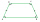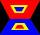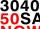# Trapezoid ABCD

ABDC is a trapezoid in which AB and CD are parallel sides measuring 6 and 9 respectively. Angles ABC and BCD are both right angles. Find the length of segment BD.

Result

x=|BD| =  0

Leave us a comment of example and its solution (i.e. if it is still somewhat unclear...):

Showing 0 comments:Be the first to comment!## Next similar examples:

1. Isosceles trapezoid v3In an isosceles trapezoid ABCD is the size of the angle β = 81° Determine size of angles α, γ and δ.
2. Rectangular trapezoidHow many inner right angles has a rectangular trapezoid?
3. Trapezium 2Trapezium has an area of 24 square cms. How many different trapeziums can be formed ?
4. HostelStudents are accommodated in 22 rooms. Rooms were 4 and 6 bed. How many rooms in which type occupied 106 children there?
5. Three digits number 2Find the number of all three-digit positive integers that can be put together from digits 1,2,3,4 and which are subject to the same time has the following conditions: on one positions is one of the numbers 1,3,4, on the place of hundreds 4 or 2.
6. Base, percents, valueBase is 344084 which is 100 %. How many percent is 384177?
7. Two circlesTwo circles with a radius 4 cm and 3 cm have a center distance 0.5cm. How many common points have these circles?
8. One-thirdA one-third of unknown number is equal to five times as great as the difference of the same unknown number and number 28. Determine the unknown number.
9. Equation?
10. Sales offCalculate what was the original price of the good, if the price after discount 25% and the subsequent rise of 20% is 400 USD.
11. CakesGrandmother baked cakes. Half of its was poppy, quarter with plum jam and 16 cheesecakes. How many cakes she baked in total?
12. RoundingThe following numbers round to the thousandth:
13. WithdrawalIf I withdrew 2/5 of my total savings and spent 7/10 of that amount. What fraction do I have in left in my savings?
14. Write 2Write 791 thousandths as fraction in expanded form.
15. Conference148 is the total number of employees. The conference was attended by 22 employees. How much is it in percent?
16. Apples 2James has 13 apples. He has 30 percent more apples than Sam. How many apples has Sam?
17. Simplify 2Simplify expression: 5ab-7+3ba-9